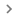# TO_DATE

Converts a provided number to a date.

### Sample Usage

`TO_DATE(25405)`

`TO_DATE(A2)`

`TO_DATE(40826.4375)`

### Syntax

`TO_DATE(value)`

• `value` - The argument or reference to a cell to be converted to a date.

• If `value` is a number or a reference to a cell containing a numeric value, `TO_DATE` returns `value` converted to a date, interpreting `value` as number of days since December 30, 1899.

1. Negative values are interpreted as days before this date, and fractional values indicate time of day past midnight.
• If `value` is not a number or a reference to a cell containing a numeric value, `TO_DATE` returns `value` without modification.

### Notes

• `TO_DATE` does not autoconvert number formats in the same way as direct entry into cells. Therefore, `TO_DATE(10/10/2000)` is interpreted as `TO_DATE(0.0005)`, the quotient of 10 divided by 10 divided by 2000.

• `TO_DATE` is not as commonly used as `DATE`, which takes a year, month, and day in numeric format as inputs.

• `TO_DATE` is the inverse of `N` as applied to a date, and equivalent to applying FormatNumberDate time from the menu bar.

`DATE`: Converts a year, month, and day into a date.

`TO_TEXT`: Converts a provided numeric value to a text value.

`TO_PURE_NUMBER`: Converts a provided date/time, percentage, currency or other formatted numeric value to a pure number without formatting.

`TO_PERCENT`: Converts a provided number to a percentage.

`TO_DOLLARS`: Converts a provided number to a dollar value.

`N`: Returns the argument provided as a number.